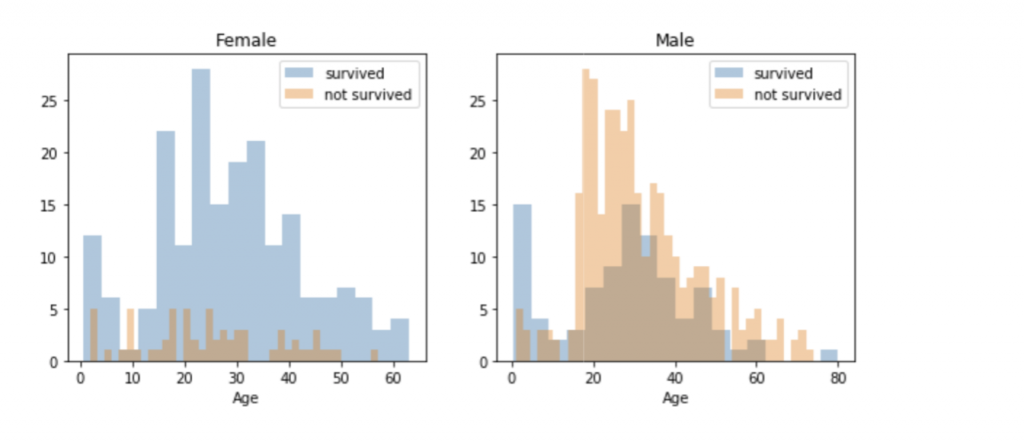# Machine Learning & Artificial Intelligence what for?

Machine Learning & Artificial Intelligence what for?

In machine learning, our goal is either prediction or clustering from Big Data. Prediction is a process where, from a set of input variables, we estimate the value of an output variable. For example, using a set of characteristics of a house, we can predict its sale price. Prediction problems are divided into two main categories:
Regression problems, where the variable to predict is numerical (e.g., the price of a house).

Classification problems, where the variable to predict is part of one of some number of pre-defined categories, which can be as simple as “yes” or “no.” (for example, predict whether a certain piece of equipment will experience a mechanical failure)

The most prominent and common algorithms used in machine learning historically and today come in three groups: linear models, tree-based models, and neural networks.

If we analyze the case of titanic as a study of survival by passengers, we can make an application of a learning system that allows us to understand who was more likely to survive.You can see that men have a high probability of survival when they are between 18 and 30 years old, which is also a little bit true for women but not fully. For women the survival chances are higher between 14 and 40.

For men the probability of survival is very low between the age of 5 and 18, but that isn’t true for women. Another thing to note is that infants also have a little bit higher probability of survival.

In the case of minors, they also had a high percentage of survival.

In the same way, learning algorithms can be applied to understand behaviors or facts and thus be able to predict behaviors or results, based on the historical data stored in our data set.